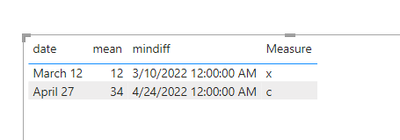cancel
Showing results for
Did you mean:Helper IV

## find latest nonblankvalue and override with text

Hi,

I have been struggling for a while with this one.

So I have a table with mean, std and date. The issue is that I need to lookup where there are values for mean the date and see if for date or the earlier date which is not blank and override the null in std with the new text value.

So for example for the first one on 10 may 17 there is a mean and i need to override the null in std, when i check in the table i see on 10 may 17 there is a null for std and the first nonblankvalue that is before 10 may is 9 may 17 with y. The null in the table for std has to be overwritten with y. How can I do that?The file can be found here:

file/Override latest nonblankvalues with text values.pbix at master · userdata21/file (github.com)

1 ACCEPTED SOLUTIONCommunity Support

Thanks for reaching out to us.

create the measures below,

``````mindiff =
var _v1=CALCULATE(MAX(Table2[date]),FILTER(ALL(Table2),Table2[date]<MIN(Table1[date])))
var _v2=CALCULATE(MIN(Table2[date]),FILTER(ALL(Table2),Table2[date]>MIN(Table1[date])))
return SWITCH(TRUE(),
ISBLANK(_v2)&&_v1<>BLANK(),_v1,
ISBLANK(_v1)&&_v2<>BLANK(),_v2,
datediff(_v1,MIN(Table1[date]),DAY) <datediff(MIN(Table1[date]),_v2,DAY),_v1,
_v2) ``````
``````Measure =
CALCULATE(MAX(Table2[std]),FILTER(ALL(Table2),Table2[date]=[mindiff]))
``````Best Regards,

Community Support Team _Tang

If this post helps, please consider Accept it as the solution to help the other members find it more quickly.

7 REPLIES 7Community Support

Thanks for reaching out to us.

create the measures below,

``````mindiff =
var _v1=CALCULATE(MAX(Table2[date]),FILTER(ALL(Table2),Table2[date]<MIN(Table1[date])))
var _v2=CALCULATE(MIN(Table2[date]),FILTER(ALL(Table2),Table2[date]>MIN(Table1[date])))
return SWITCH(TRUE(),
ISBLANK(_v2)&&_v1<>BLANK(),_v1,
ISBLANK(_v1)&&_v2<>BLANK(),_v2,
datediff(_v1,MIN(Table1[date]),DAY) <datediff(MIN(Table1[date]),_v2,DAY),_v1,
_v2) ``````
``````Measure =
CALCULATE(MAX(Table2[std]),FILTER(ALL(Table2),Table2[date]=[mindiff]))
``````Best Regards,

Community Support Team _Tang

If this post helps, please consider Accept it as the solution to help the other members find it more quickly.Helper IV

it is not about finding the first value or latest value and filling it with that value, but i need to dynmically check when date and mean has a value and lookthat value up ifor std where there was a value on that day or ealier and take the first with each row.Helper IV

date |  mean                                                                  date | std

27.4     34                                                                        24.4    c

12.3     12                                                                        12.3   blank

10.3 x

9.3  b

output i want to see:

date |  mean  | std

27.4     34        c

12.3     12        x

there are two different table 1 and 2 and what i need to do is lookup if date in first table is 12.3 then i need to check in table 2 if there is a std for 12.3 if not i have to take the latest value that is not blank, in this case it would be x.

How would I do that in DAXHelper IV

@daXtreme I do not fill in the blanks with backward values. I think maybe I have not explained it very well. But what i have is i have 3 columns, date, mean and everytime there is a mean that is blank is where we have values for std. What I need to do is check the date for when there is a mean lets say 7 august there is a value for mean and then in the same date column i have to check where std is not blank and then find the first instance on that date or before the value for std and put that value in there. So in std column the earlier date there is a value might be 5 august which is c for example. Then in the table it has to say c next to mean and dateHelper IV

Hi @daXtreme  i do not need to fill in the blanks. I have to lookup the dates and check the latest value before the date where mean has a value and look up with std for that date or the latest date before ther is a value.Super User

As I said, Power Query has a special method for this: filling blanks with forward/backward values.Super User

OK, why can't you use Power Query to fill in the blanks? It's a one-click operation.